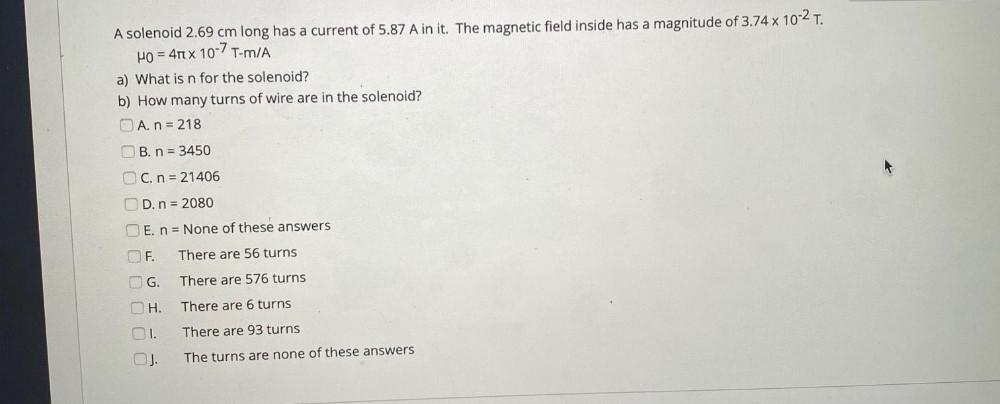Question:

# A solenoid 2.69 cm long has a current of 5.87 A in it. The magnetic field inside has a magnitude of 3.74 x 10-2 T. H0 = 4 x 10-7A solenoid 2.69 cm long has a current of 5.87 A in it. The magnetic field inside has a magnitude of 3.74 x 10-2 T. H0 = 4 x 10-7T-mA a) What is n for the solenoid? b) How many turns of wire are in the solenoid? O A. n = 218 OB. n = 3450 OC. n = 21406 OD. n = 2080 E. n = None of these answers F. There are 56 turns OG. There are 576 turns OH. There are 6 turns 1. There are 93 turns The turns are none of these answers